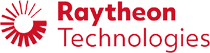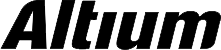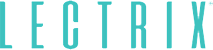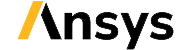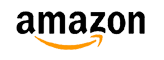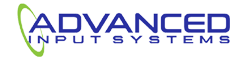﻿ How To Run a PCB Impedance Calculation Without a Field Solver | NWES Blog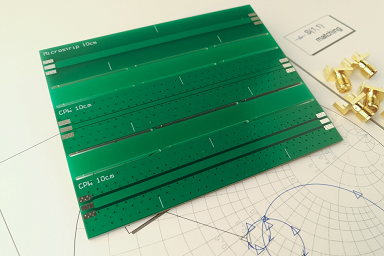## How To Run a PCB Impedance Calculation Without a Field Solver

By

A PCB impedance calculation generally refers to the task of calculating trace impedance, or transmission line impedance, based on the stackup and material configuration used in your design. It is widely accepted that the most accurate impedance calculations are produced with a 3D field solver due to the complex geometry in any PCB layout. However, you can get a very accurate calculation without the use of advanced field solver software.

Although the geometry of a PCB layout may be complex, the routing features in ECAD software require a determination of trace impedance, especially for high speed and high frequency signals. The reason for this is the need to enforce a specific trace geometry during routing, rather than to interactively check for things like parasitics. Not all signals or boards will need impedance control, but for those that do, a calculation of the trace impedance is needed on the front end while planning the pcb stackup.

To get started calculating impedance, it's important to know different models and how calculator applications work. Not all of these are based on field solvers. In this article, we'll discuss these points and I'll discuss when these calculators will produce less-than-accurate results. We won't look at PDN impedance in this article as the front-end portion of the design is more the domain of a spice simulation, while evaluating the layout requires a field solver and correlation with measurements. Instead, we’ll look at the fundamental models used in transmission line impedance and how they are constructed.

## PCB Impedance Calculations and Models

Before you start calculating impedances and setting up design rules, you will need to decide where and how you are routing your traces, as well as the stackup configuration. At this point, we can assume you already know which interfaces are needed in your circuit board and your components. Once that is known and you've determined the single ended and/or differential impedance specifications in your design, you can start designing the stack up and calculating trace impedance. Trace impedance must be determined and finalized while designing the stack up because any later change in the layer stack will require a change in the trace width that produces your target impedance.

### IPC-2141

Just about every calculator you find speak calculator you find online will implement implement a trace impedance calculation used it's calculation using the IPC-2141 model. The trace impedance equations for microstrips and symmetric striplines specified in IPC-2141 is shown below.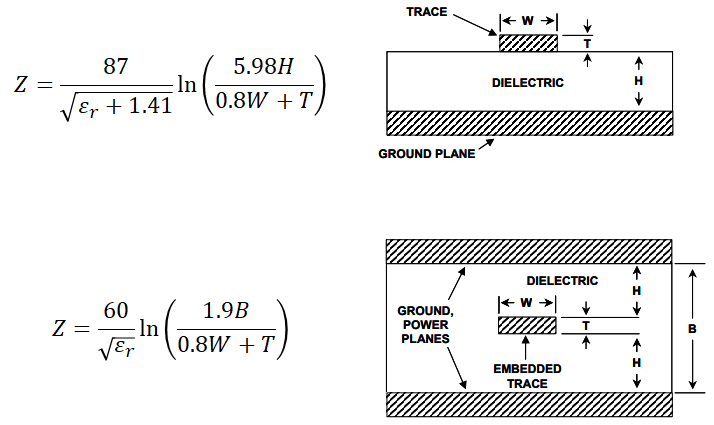These two equations from the IPC-2141 are used to get the characteristic impedance of striplines and microstrips. These equations are only accurate near 50 Ohm target impedance.

Note that there is an additional phenomenological equation defining differential impedance, which can then be halved to get the odd-mode impedance of a single trace.

This model was originally developed by analyzing trace impedance measurements using a regression model. These measurements were gathered from traces that targeted a 50 Ohm impedance. Therefore, the IPC-2141 equations are only accurate when attempting to calculate a trace width corresponding to a 50 Ohm single-ended impedance.

It is well known that the IPC-2141 models yield inaccurate results when calculating the width required to produce any impedance that deviates from a 50 Ohm target. This should not be surprising because the equations are a regression model based only on data from a 50 Ohm target. Because impedance is generally a nonlinear function of geometry, it should not be surprising that a regression model targeting 50 Ohm impedance will not easily extrapolate to other impedance values. This model will yield inaccurate results and excessive S11 values when targeting higher or lower impedance; two examples would be a single ended trace in a DDR link or classic LVDS.

Of all the available analytical models for calculating PCB trace impedance, Wadell's equations are arguably the most accurate. These equations can be found in Wadell's seminal textbook, Transmission Line Design Handbook. This textbook contains a huge number of number of equations for various transmission line geometries, both on and off a PCB. Some of the available pcb trace arrangements include:

• Single-ended microstrips and striplines
• Even and odd mode impedances for coupled lines
• Coplanar lines
• Asymmetric lines

Although these models produce highly accurate results when compared with measurements, the equations are difficult to work with if you are trying to calculate a trace width that produces a target impedance. Some of these models include dozens of parameters that each have their own equations, so they are difficult to use by hand. These impedance equations are nonlinear functions of geometry that can't be solved for one of the geometric parameters or a ratio of parameters. The result is that, unlike the IPC-2141 models, determining width for a target impedance requires solving a transcendental equation. The resulting transcendental solution would actually be solved in terms of a trace-to-substrate thickness ratio for a single-ended trace, as well as a trace width to trace spacing ratio for differential pairs.

For this reason, designers will typically take two approaches to determine trace impedance from Wadell’s equations:

1. Trial and error: Online PCB impedance calculators that Implement Wadell’s equations do not calculate the trace width and/or spacing directly. Instead, they force you to use trial and error to figure out the best geometry that produces your target impedance.

2. Random or directed search: This is a form of trial-and-error that is implemented in a script or application. Evolutionary or genetic algorithms are most popular for this type of calculation, although machine learning approaches can be used to speed up a directed search algorithm.

To see how we implement Wadell’s equations in our PCB impedance calculation tools, take a look at our impedance calculator page. We've created several calculators that can be used to determine trace impedance while accounting for copper foil roughness and loss tangent in the PCB dielectric.

### Machine learning and AI

The most sophisticated methods for determining impedance in a PCB, whether for low impedance structures like a PDN or standard impedance structures like interconnects, will use AI and machine learning techniques. Machine learning as applied to interconnect design requires a set of data that is used to learn trends that determine a target performance or another signal integrity metric. The machine learning model can then adjust the trace geometry values and eventually converge to the desired performance.

Obviously, the performance must be feasible within the loss and dispersion limits of the desired interconnect. However these models can be generalized to cases where data on parasitics from nearby structures or very unique structures without analytical solutions are involved. In these cases, the machine learning model will be trained against an array of field solver results or measurements. Some example results showing this convergence for a long interconnect involving two packages, multiple vias, and AC coupling caps is shown below.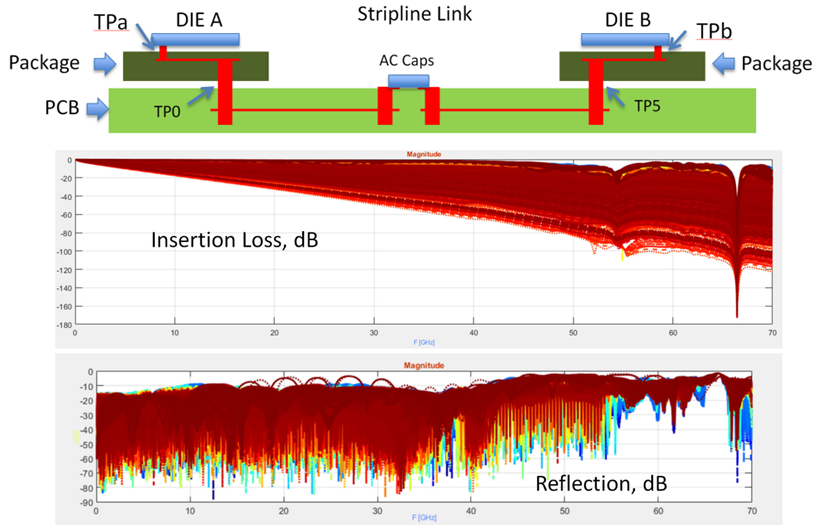Image and data source: Manukovsky, A., et al. "Machine Learning Applications for COM Based Simulation of 112Gbs Systems." DesignCon 2020, (January 29, 2019).

## Summary

Once the impedance of an interconnect, or at least the structures in an interconnect, are known, the designer can use the results to determine other important signal Integrity metrics. These other metrics might include:

• S parameters or other network parameter sets
• A transfer function and its impulse response function
• A simulated TDR response
• Input impedances in cascaded networks
• Eye diagrams

Before you start fumbling with online trace impedance calculators for advanced designs, look to a more sophisticated PCB impedance calculation method that does not require a field solver. Once the PCB layout is complete, the use of a field solver becomes appropriate to evaluate and qualify the layout, as well as to ensure parasitics have not created significant deviations from the target impedance.

At NWES, we provide PCB design and layout services to SMBs, large enterprises, and aerospace and defense electronics companies. Our PCB impedance calculation methods are among the most cutting-edge solutions that do not require applying a field solver to a lossy interconnect. We’ve also partnered directly with EDA companies and multiple ITAR PCB manufacturing firms, and we help our clients get through the PCB manufacturing process with ease. Contact NWES for a consultation.

* indicates required

## Our Clients and Partners